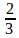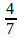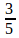## Important Partnership for SBI PO – 15

1) A, B and C started a business. They invest Rs.20,000, Rs.40,000 and Rs.60,000 respectively. At the end of the first year, B withdraws Rs.15,000, while at the end of the second year, C withdraws Rs.15,000. In what ratio will the profit be shared at the end of 3 years?

a) 18 : 19 : 2

b) 4 : 6 : 11

c) 19 : 2 : 18

d) 11 : 18 : 19

e) None of these

b) 4 : 6 : 11

A invests Rs.20000 for 36 months

B invests Rs.40000 for 12 months & Rs.25000 for 24 months

C invests Rs.60000 for 24 months & Rs.45000 for 12 months

Ratio of their profits, A : B : C is,

20000×36 : 40000×12 + 25000×24 : 60000×24 + 45000×12 = 4 : 6 : 11

2) 3 partners X, Y & Z start a business. Thrice of X’s capital is equal to five times of Y’s capital and Y’s capital is seven times Z’s capital. If Y’s profit is Rs.6,300. Then find the total profit.

a) Rs.17000

b) Rs.17700

c) Rs.18000

d) Rs.18800

e) Rs.19000

b) Rs.17700

Let the capital of Z be m

Then the capital of Y is 7m

Then the capital of X is 35m/3

Ratio of their profit is,

X : Y : Z = 35m/3 : 7m : m = 35 : 21 : 3

Total profit =6300/21 ×59 = Rs.17,700

3) Ram and Ramesh are partners in a business. Ram contributes 1/5th of the total capital for 18 months and Ramesh received 2/5th of the profit. Then how long Ramesh invest the money in the business?

a) 8 months

b) 6 months

c) 5 months

d) 3 months

e) None of these

d) 3 months

Let the total profit be X

Then Ramesh’s profit is 2/5 X

Also, Ram’s profit is 3/5 X

Ratio of Ram & Ramesh profit =3/5 X :2/5 X = 3 : 2

Let the total investment be P

Ram’s investment =1/5×P (for 15 months)

Then Ramesh’s investment =4/5×P

Ratio of Ram & Ramesh profit = 3 : 2 =1/5×P×15 :4/5×P×T

T = 3 months = Ramesh’s investment time

4) Three partners P, Q & R shared a profit in the ratio 6:8:9. They had partnered for 10 months,8 months and 7 months. What was the ratio of their investments?

a) 32 : 7 : 9

b) 42 : 8 : 11

c) 42 : 7 : 9

d) 41 : 7 : 9

e) None of these

c) 42 : 7 : 9

Let the investment of A, B & C be X, Y & Z respectively.

Ratio of P,Q & R profit = 6 : 8 : 9 = 10X : 8Y : 7Z

• 6/8=10X/8Y=> Y =5/3 X

6/9=10X/7Z => Z =15/7 X

Then ratio of their profit = 10X :5/3 X :15/7 X = 42 : 7 : 9

5) A invested 15% more than B’s investment. B invested 20% less than investment of C. If the total investment of A, B & C is Rs.12240. Then find average investment of both A & C?

a) Rs.4240

b) Rs.4320

c) Rs.4400

d) Rs.4700

e) None of these

b) Rs.4320

Let the investment of C be 100%.

Then B’s investment is 80% & also A’s investment is 92%.

Total investment A, B & C is 272% = 12240 => 1% = 45

Total investment of A & C is 192% = 45×192 = Rs.8640

Average investment of A & C =8640/2 = Rs.4320

6) A, B, & C started a business with their investment in the ratio::, after 6 months A has withdrawn half of his investment. Also, A will get 25% of profit for being an active partner. At the end of a year total profit earned was Rs.37,440. Then find the total profit earned by B & C,

a) Rs.20200

b) Rs.18500

c) Rs.19680

d) Rs.17840

e) None of these

c) Rs.19680

Let the investment of A, B & C be 2/3 X, 4/7 X & 3/5 X respectively. (i.e)70X, 60X & 63X respectively.

Profit ratio of A, B & C is,70X×6 + 35X×6 : 60X×12 : 63X×12 = 35 : 40 : 42

For being active partner A’s commission = 37440×25/100 = Rs.9360

Remaining profit = 37440 – 9360 =Rs.28080.

Total profit earned by B & C = 28080×82/117 = Rs.19680

7) X started a business with Rs.42,000 and is later joined by Y with Rs.72,000. After how many months did Y join if the profits after 3 yrs divided in the ratio 8 : 7 for Y & X respectively?

a) 8 months

b) 9 months

c) 11 months

d) 12 months

e) None of these

d) 12 months

Let the number of months invested by Y be T

Ratio of X & Y after 3yrs is,

7/8 = ( 42000×36)/(72000×T)=>X=24 months

Number of months after which Y joined = 36 – 24 = 12 months

8) Ankita and Bhanu are partners in a business. Ankita contributes 4/7th of the capital for 18 months and Bhanu received 2/5th of the profit, for how long Bhanu money was used?

a) 16 months

b) 17 months

c) 18 months

d) 20 months

e) None of these

a) 16 months

Let the total profit earned be X.

Profit earned by Bhanu =2/5 × X

Profit earned by Ankita =3/5 × X

Ratio of Ankita & Bhanu profit =3/5×X :2/5×X = 3 : 2

Let the total capital investment be P

Then Ankita contributes 4/7 th of P for 18 months & Bhanu contributes 3/7 th of P for Y (assume)months.

Then ratio of profit Ankita & Bhanu is,

3/2 = ( 4/7 ×P ×18)/(3/7 ×P ×Y) => Y = 16 months = Bhanu’s money used.

9) Ramu being the active partner receives 2/7th of profit and the remaining is divided between Ravi and Ramesh in the ratio of 3:2. If the difference between the profit shares of Ravi and Ramu is Rs.2700.What is Ramesh’s share in Rs?

a) Rs.5400

b) Rs.6000

c) Rs.6600

d) Rs.7000

e) None of these.

a) Rs.5400

Let the total profit be X.

Then Ramu’s share =2/7 × X

Remaining share =5/7 × X

Share of Ravi =5/7 × X ×3/5 =3/7 × X

Difference of Ravi & Ramu share,

3/7 × X –2/7 × X = 2700 => X = Rs.18900

Share of Ramesh =5/7 × 18900 ×2/5 = Rs.5400

10) A, B and C enter into a partnership Business with A’s contribution Rs.39000. If out of a total profit of Rs.2700, A gets Rs.1300 and B gets Rs.900, C gets Rs.500 then total capital of B & C is,

a) Rs.36000

b) Rs.44000

c) Rs.42000

d) Rs.40000

e) None of these